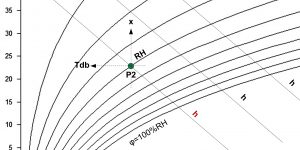# Enthalpy of wet air

Enthalpy: h [kJ/kg]

The enthalpy h (or heat content) of wet air consists of the enthalpy of dry air and the enthalpy of water vapor.
If the air is in contact with a damp surface or we spray water into it (for example in an air washer), the water evaporates and at the same time removes the heat of evaporation exclusively from the resulting mixture. Since there is practically no exchange of energy with the environment during this process, the enthalpy of the air-water mixture does not change and the state change takes place with a constant enthalpy.

However, there is a decrease in the appreciable and increase in the latent proportion of the heat content in the air. This shift causes the mixture to cool. The slope of the curves with constant enthalpy in the h-x diagram (so-called isoentalp or adiabat) is given by the ratio of sensible and latent heat. Assuming that in the construction of isotherms we respect the different specific heat of dry and wet air, the isoentalps (curves with constant enthalpy) run in parallel with each other. The enthalpy scale is usually shown below the saturation curve in the h-x diagram.

h= Tdb * (1.01 + 0.00189 * x) + 2.5 * x